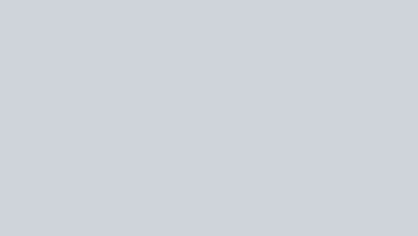In the below fig. lines AB. CD and EF intersect at O. Find the measures of ∠AOC, ∠COF, ∠DOE and ∠BOF.
Question:

In the below fig. lines AB. CD and EF intersect at O. Find the measures of ∠AOC, ∠COF, ∠DOE and ∠BOF.Solution:

AOE and EOB are linear pair of angles

∠AOE + ∠EOB = 180°

∠AOE + ∠DOE + ∠BOD = 180°

⟹ DOE = 180 – 40 – 35 = 105

Vertically opposite side angles are equal

DOE = COF =105

Now, ∠AOE + ∠AOF = 180°

[Linear pair]

AOE + AOC + COF = 180

⟹ 40 + AOC +105 = 180

⟹ AOC = 180 – 145

⟹ AOC = 35

Also, BOF = AOE = 40 (Vertically opposite angles are equal)